# 关系数据理论

## ⭐️数据依赖

### 函数依赖

#### 函数依赖相关术语

• $X \rightarrow Y$，则称$X$为这个函数依赖的决定属性组，又称决定因素，读作$X$决定$Y$。 ⚠️X、Y是属性或者属性集，$X \rightarrow Y$$Y$依赖$X$$X$决定$Y$

• $X \rightarrow Y$，但$Y \nsubseteq X$，则称$X \rightarrow Y$非平凡的函数依赖。 ⚠️$X \subseteq Y$$Y$包含$X$

• $X \rightarrow Y$，但$Y \subseteq X$，则称$X \rightarrow Y$平凡的函数依赖。对于任一关系模式，平凡的函数依赖均必然成立，不反映新的语义（没什么用，一般不考虑）。

• ⭐️在$R(U)$中，如果$X \rightarrow Y$，并且对于$X$的任何一个真子集$X^{'}$，都有$X^{'} \nrightarrow Y$，则称$Y$$X$完全函数依赖，记作$X\stackrel{F}{\longrightarrow}Y$。 💡只有$X$整个集合才能决定$Y$成为完全函数依赖，存在$X$中的一子属性决定$Y$称为部分函数依赖。

• ⭐️同理，若$X \rightarrow Y$，但$Y$不完全依赖于$X$，则称**$Y$$X$部分函数依赖**，记作$X\stackrel{P}{\longrightarrow}Y$
• $R(U)$中，如果$X \rightarrow Y$$Y \rightarrow Z$$Y \nrightarrow X, Y \nsubseteq X,Z \nsubseteq Y$），则称Z对X传递函数依赖，记作$X\stackrel{传递}{\longrightarrow}Y$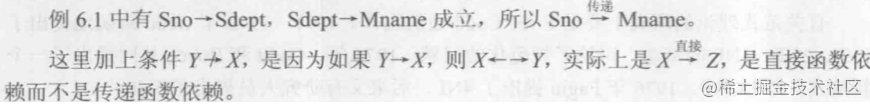这个地方的概念必须得看懂。

#### 码的函数依赖定义

• $K$$R$中的属性火属性组合，若$K \stackrel{F}{\longrightarrow} U$，即$U$完全函数依赖于$K$，则称$K$$R$候选码若候选码多于一个，选定其中一个为主码
• 如果$U$部分函数依赖于$K$，则称$K$超码。候选码是最小的超码，即$K$的任一真子集都不是候选码。
• 包含在候选码中的属性成为主属性，否则称为非主属性。整个属性组全是码，称为全码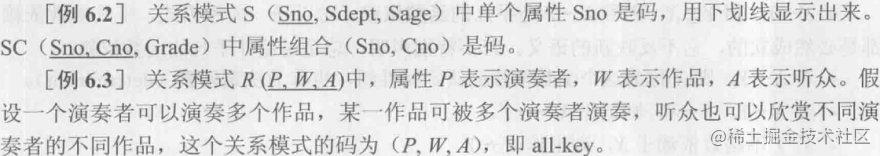# 范式与规范化

## 第二范式

$R∈1NF$，且每一个非主属性==完全函数依赖==于任何一个候选码，则称为第二范式，记作$R∈2NF$。 2NF通过消除部分函数依赖，可以在一定程度上减轻1NF中存在的插入异常、删除异常、数据冗余大、修改复杂等问题，但并不能完全消除关系模式的各种异常情况和数据冗余。

## 第三范式（3NF）

### 2NF回顾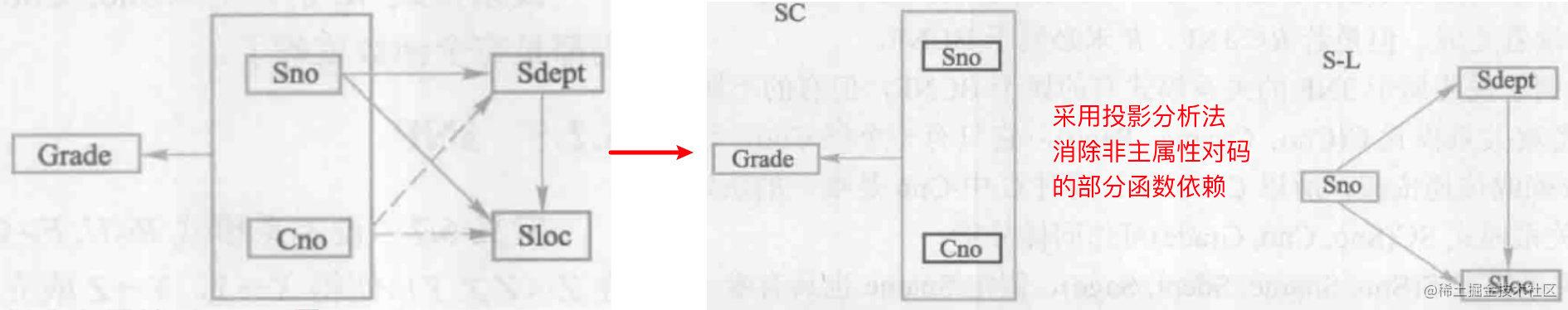💡简单来说就是再拆分几张表出来，让表中只剩下完全依赖，部分依赖是一切问题的根源。

### 2NF中的问题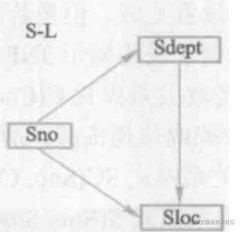1. 插入异常 如果某个系暂时没有在校学生，我们就无法把这个系的信息存入数据库。

2. 删除异常 如果某个系的学生全部毕业了，我们在删除该系全部学生信息的同时，就把这个系的信息也丢掉了。

3. 数据冗余度大 每一个系的学生都住在同一个地方，按理来说只存一次就行了，但关于在每个学生信息里，该系的住处的信息却重复出现。

4. 修改复杂 学校调整学生住处时，由于刚刚提到的关于每个系的住处信息是重复存储的，修改时必须同时更新该系所有学生的$Sloc$属性值。

### 问题原因

$S-L$$Sloc$传递函数依赖于$Sno$， 即非主属性传递函数依赖码。 💡可见非主依赖这个东西是一切问题的根源。

### 2NF问题的解决办法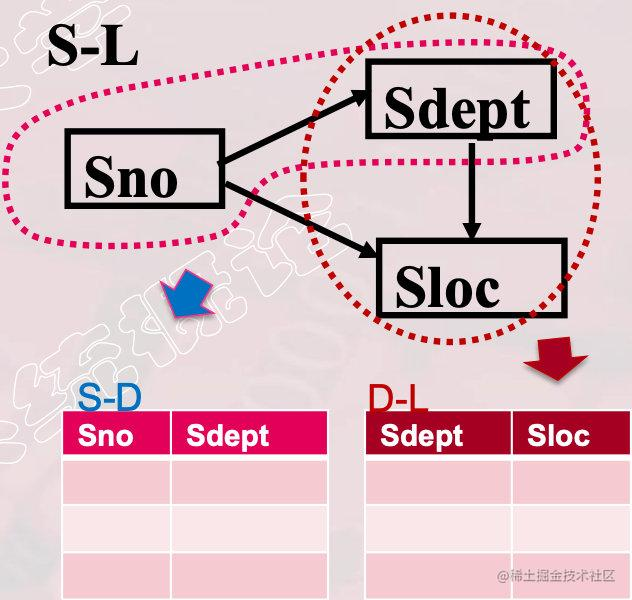### ⭐️3NF关系模式的性质

• $R\in3NF$，则**$R$的每一个非主属性既不部分函数依赖于候选码也不传递函数依赖于候选码**。
• $R\in3NF$，则$R\in2NF$
• 采用投影分解法将一个$2NF$的关系分解为多个$3NF$的关系，可以在一 定程度上解决原$2NF$关系中存在的插入异常、删除异常、冗余度、修改复杂等问题。但依然并不能完全消除关系模式中的各种异常情况和数据冗余。

## BC范式（BCNF）

### 引入实例

1. 假设每一教师只教一门课：$T→J$

2. 假设每门课只由一个教师教，学生选定某门课，就能确定了一个固定的教师：$（S，J）→T$

1. 学生选修某个教师的课就确定了所选课的名称： $(S，T)\rightarrow J$

⚠️这里我们一直都在用假设化简情况，自己仔细思考一下，可想而知现实世界中这样的依赖关系有多么复杂，这也就是范式这个东西的重要性所在。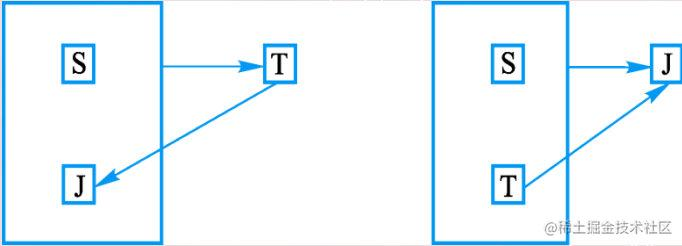### 3NF中的问题

1. 插入异常 如果某个教师开设了某门课程，但尚未有学生选修，则有关信息也无法存入数据库中。
2. 删除异常 如果选修过某门课程的学生全部毕业了，在删除这些学生元组的同时，相应教师开设该门课程的信息也同时丢掉了。
3. 数据冗余度大 虽然一个教师只教一门课，但每个选修该教师该门课程的学生元组都要记录这一信息。
4. 修改复杂 某个教师开设的某门课程改名后，所有选修了该教师该门课程的学生元组都要进行相应修改。

### 解决办法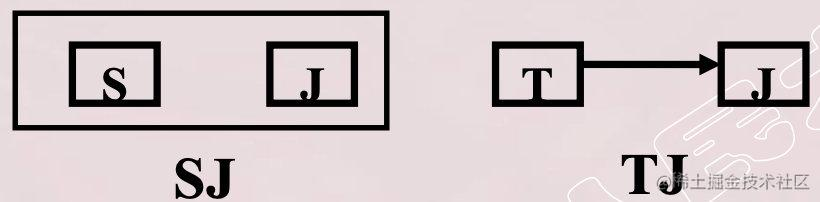### BCNF关系模式的性质

1. 所有非主属性对每一个码都是完全函数依赖。
2. 所有主属性对每一个不包含它的码也是完全函数依赖。
3. 没有任何属性完全函数依赖于非码的任何一组属性。

💡全是完全依赖，全是码就完事了。

## 规范化总结

• 一个关系模式只要其分量都是不可分的数据项，它就是规范化的关系模式，但这只是最基本的规范化。
• 规范化程度过低的关系模式不一定能够很好地描述现实世界，可能会存在插入异常、删除异常、修改复杂、数据冗余等问题，解决方法就 是对其进行规范化，转换成高级范式。
• 一个低一级范式的关系模式，通过模式分解可以转换为若干个高一级范式的关系模式集合，这种过程就叫关系模式的规范化
• 关系数据库的规范化理论是数据库逻辑设计的工具。
• 高级的关系模型必然包含低级的关系模型。

### 关系模型规范化基本步骤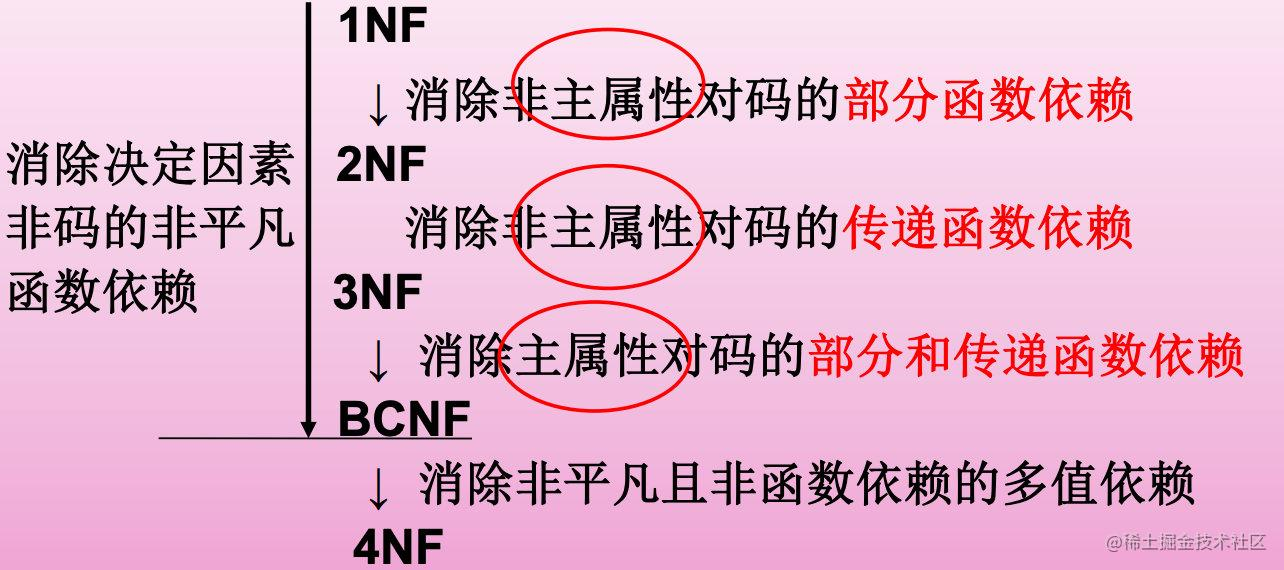### 规范化的基本思想

• 逐步消除数据依赖中不合适的部分，使模式中的各关系模式达到某种程度的“分离”
• 采用“一事一地”的模式设计原则 让一个关系只描述一个概念、一个实体或者实体间的一种联系，若多于一个概念，就把它“分离”出去。
• 规范化实质上是概念的单一化

# 模式分解

## 模式分解的抽象定义

$R$的一个分解是指：$\rho={R_1, …, R_n}$， 其中$U=U_1\cup U_2\cup ···\cup U_n$，并且没有$U_i ⊆ U_J$$1 ≤ i$$j ≤ n$$F_i$$F$$U_i$ 上的投影。 💡可以看到R, U被分解成了若干个小部分。 相应地将$R$存储在二维表$r$中的数据分散到二维表 $r_1，r_2，… , r_n$中去，其中$r_i$$r$在属性集$U_i$上的投影。

## 模式分解三条准则

• 分解具有无损连接性
• 分解要保持原有的函数依赖
• 分解既要保持函数依赖，又要具有无损连接性。

### 模式分解实例#### 正确的模式分解

$S-L$分解为二个关系模式： $ND(Sno, Sdept),Sno→Sdept$

$DL(Sdept, Sloc),Sdept→Sloc$ 这种分解不仅保持函数依赖性, 还具有无损连接性。 💡用正常数据库开发的思维来考虑，也只能这样分。

#### 错误的模式分解

• 既不具有无损连接性，也未保持函数依赖。 例如很极端的直接分解为3个关系模式$SN(Sno),SD(Sdept),SO(Sloc)$

• 不具有无损连接性，但保持函数依赖。 例如分解为$NL(Sno, Sloc),DL(Sdept, Sloc)$$NL$$DL$自然连接的结果为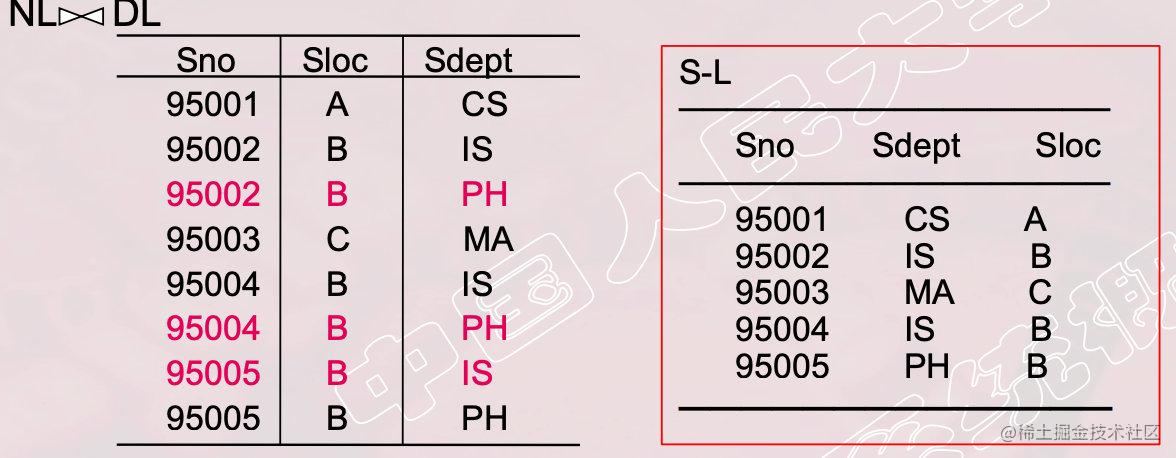$NL⋈DL$比原来的$S-L$关系多了三个元组，则无法知道原来的$S-L$关系中究竟有哪些元组， 即此分解丢失了信息。 ⚠️IS和PH系宿舍楼都在B导致的。

• 具有无损连接性，但未保持函数依赖。 例如分解为$ND(Sno, Sdept),NL(Sno, Sloc)$，没有考虑原函数依赖$Sdept→Sloc$